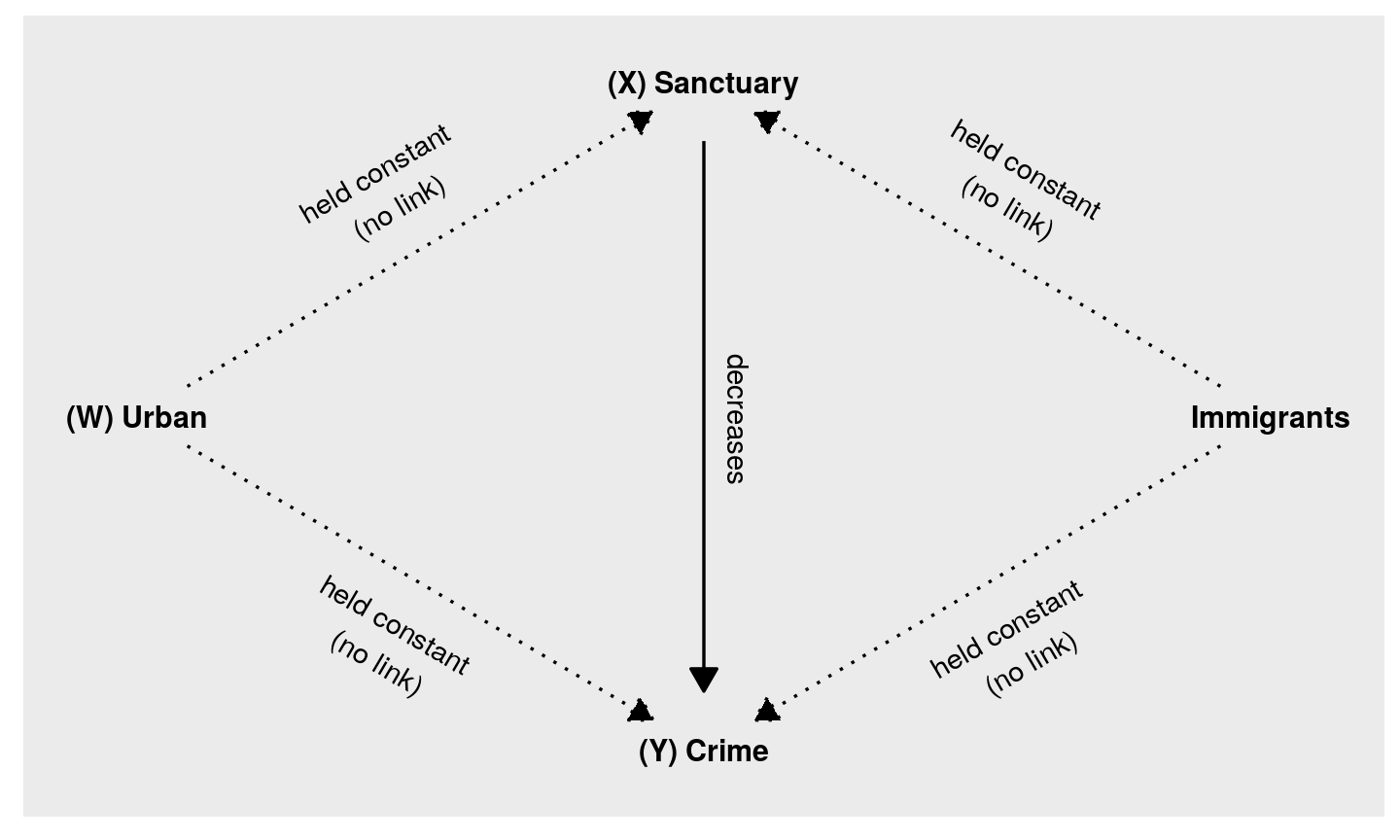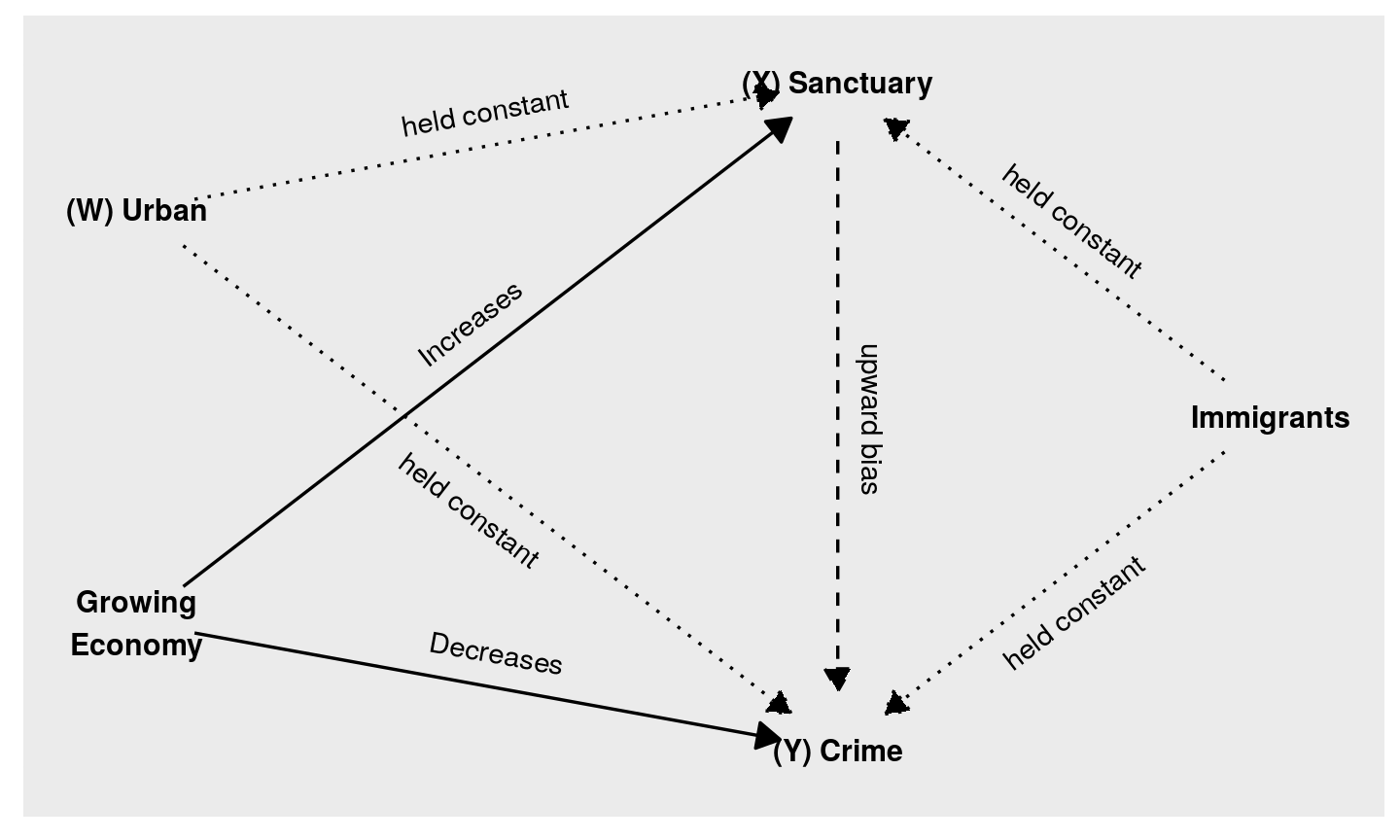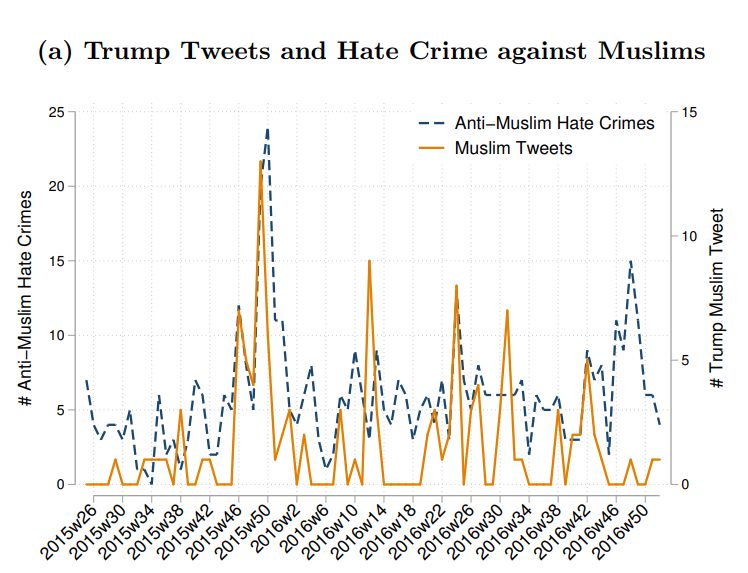March 22, 2019

## Plan for Today:

### (1) Solutions for Bias

• adjustment-based
• conditioning
• what is it?
• how does it work?
• assumptions!

## Solutions to Bias

### adjustment-based

• Identify possible confounding variables (e.g. $$W, Z, V, U$$)
• Measure these variables
• adjust correlation of $$X$$ and $$Y$$ by "conditioning" on confounding variables

### design-based

• Compare cases that, by assumption, are
• similar in terms of confounding variables $$W$$/ potential outcomes of $$Y$$
• exposed to $$X$$ in a manner unrelated to $$W$$/potential outcomes of $$Y$$

## Conditioning

A more general approach to the comparative method is to adjust using:

### conditioning

when we observe $$X$$ and $$Y$$ for multiple cases, we examine the correlation of $$X$$ and $$Y$$ within groups of cases that have the same values of confounding variables $$W, Z, \ldots$$.

How does conditioning solve confounding?

• By holding $$W$$ constant in comparison, can be no relationship between $$W$$ and $$X$$, $$W$$ and $$Y$$.
• By definition, no longer confounding

## Conditioning

Sometimes we think about "conditioning" like this:

• conditioning lets us find the correlation between $$X$$ and $$Y$$, ceteris parabis, "all else being equal".

But all else does not need to be equal:

• We only need to compare cases that are the same on confounding variables
• same on variables that are causally linked to $$X$$ and $$Y$$

## Adjustment: Example

### Sanctuary Cities

• We saw in toy example, an "unadjusted" correlation between $$Sanctuary$$ and $$Crime$$ led to confounding and bias

• Conditioning on $$Urban$$ solved the confounding, removing the bias

## Adjustment: Example

### A Real Test Wong (2017)

Examines crime rates in 2492 US counties. 608 are "Sanctuary" counties.

Researcher matched counties on:

• population
• foreign-born percentage of the population
• percentage of population that is Latino/a
• level of urban development

## Adjustment: Example

### A Real Test Wong (2017)

Compared to similar non-sanctuary counties

• Crime Rate is 35.5 crimes per 10k fewer in sanctuary counties
• Difference has low $$p$$ value: unlikely to have happened by chance

Sanctuary policies do not cause an increase in crime

## Adjustment: Example## Adjustment: Example

### Can you think of any problems here?

• What else might be related to sanctuary policies?
• economic growth $$\to$$ jobs $$\to$$ educated citizens $$\to$$ sanctuary
• economic growth $$\to$$ jobs $$\to$$ lower crime## Adjustment: Assumptions

In order to infer $$X$$ causes $$Y$$ if $$X,Y$$ correlated after adjustment

### Must Assume

1. There are no other confounding variables (other than the ones you conditioned on)
• that is, you have conditioned on ALL confounding variables
• sometimes called 'ignorability assumption': you can ignore other variables

How do we know we have found and measured all confounding variables?

## Adjustment: Assumptions

In order to infer $$X$$ causes $$Y$$ if $$X,Y$$ correlated after adjustment

### Must Assume

1. Variables used to condition relationship between $$X$$ and $$Y$$ are measured without error.
• even random measurement error in confounding variables leads conditioning to fail.
• you are no longer comparing like-with-like.

## Adjustment: Limitations

In addition to assumptions: adjustment has other limitations

• We are LIMITED by how many variables we can adjust for
• more variables means: we need more cases
• less likely we find cases that exactly match
• relevant confounding variables not adjusted for

### Side note

We have shown adjustment that finds identical cases.

• in practice, researcher do not do this; use algorithms that approximate this

## Adjustment: Summary

Adjustment: "adjusts" correlation of $$X$$ (cause) and$Y$ (outcome) by conditioning on specific, potentially confounding variables

• Examine correlation of $$X,Y$$ within groups with same values for confounding variables (condition)
• We assume: within these groups, $$X$$ is as-if randomly assigned
• Or, we assume: within these groups, nothing other than $$X$$ could affect values of $$Y$$

We can infer causality ONLY IF:

• no other unobserved/unknown confounding variables
• confounding variables measured without error

## Design

design-based solutions to confounding:

• Do not identify and measure all confounding variables
• Choose a comparison that eliminates bias from many known/unknown measurable/unmeasurable confounding variables

Contrast to adjustment:

• focus less on measuring known confounding variables to find comparisons among many cases
• choose fewer cases where confounding is plausibly less or absent

## Design

### Types of designs:

Designs using conditioning

• Compare same case over time
• Compare cases known to be similar at same time
• "Differences in Differences"

Designs using random exposure to $$X$$

• experiments
• "natural experiments"

## Design

### Designs using conditioning

Choice of comparison holds many confounding variables constant

• we condition, not by finding confounding variables and measuring
• we condition by choosing cases known to be similar

Holds constant many confounding variables

## Design: Same Case

• Most similar case to a case?
• ITSELF

## Design: Same Case